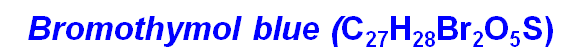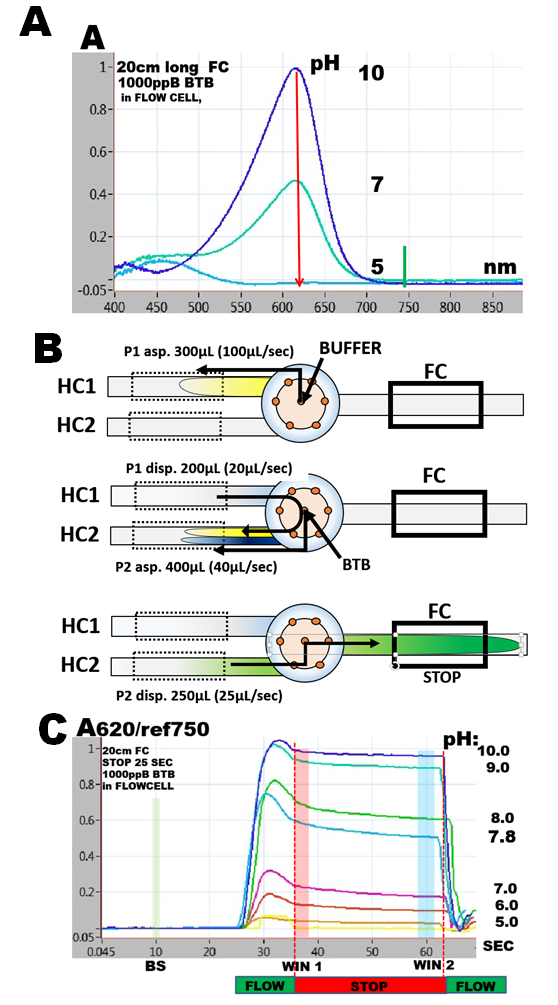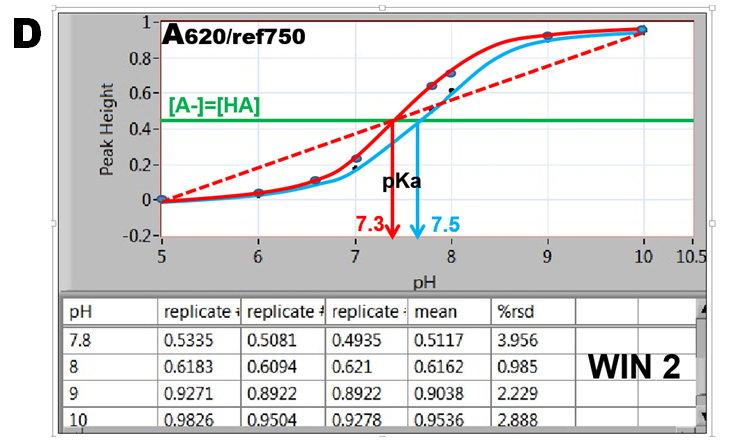Dissociation Constant of Bromothymol Blue

indicator, yellow in protonated and blue in deprotonated form with transition point between pH 7 and 8. It has a well defined spectrum with maxima at 450nm and 620nm, their absorptivity being dependent  on pH (A).
This acid/base equilibrium is described by Henderson-Hasselbach equation:
pH = pKa +log[A-]/[HA]
where pKa is dissociation constant and [A-] and [HA] are deprotonated and protonated concentrations of  BTB. The simplest approach to determination of pKa of BTB is to measure changes of absorbance  with pH at 620nm, because at this wavelength the absorbance is linearly dependent on [A-] (1.5.3. fig.D) and is only due to concentration of the deprotonated form [A-].(A). Flow programming (B) and software script are identical to the one used to determine molar absorptivity, albeit 2000 ppB BTB solution is introduced through reagent port, while buffers in the range of pH 5 to pH 10 are aspirated via sample port. DI water is used as carrier. Because the response curves show  decrease of absorbance (C) during the stop flow period,  the data were collected at its beginning (WIN1) as well as at its end (WIN2) .

1.5.5.By plotting absorbance versus pH values the S-shaped indicator curves are obtained revealing pH=pK values located at inflection point where [A-]=[HA].(D). The data collected in WIN 2 result in the blue curve, while data obtained in WIN 1 are shown as red curve. Furthermore, since absorbance A at 620nm is a linear function of  [A-] (1.5.3. fig. D.), the red dotted line connecting fully deprotonated (pH=10) and protonated BTB (pH=5) intersects at A/2 at pH=pKa. The red S-curve and the linear extrapolation intersect at the same pH yielding pKa= 7.3. The listed value of BTB dissociation constant (Bishop 1978) is pKa=7.3. The blue S curve yields pKa=7.5. The reason for decrease of absorbance during stop flow period is presently unknown.

E. Bishop Indicators Pergamon Press Oxford 1978. AlsoCRC Handbook of Chemistry and Physics; Lide, D. R., Ed.; CRC Press:: Boca Raton, FL, 2007-2008

is an acid base